## Prisms and their Applications.

### Introduction

A prism is one or several blocks of glass of glass, through which light passes and refracts and reflects off its straight surfaces. Prisms are used in two fundamentally different ways. One is changing the orientation, location, etc. of an image or its parts, and another is dispersing light as in a refractometers and spectrographic equipment. This project will only deal with the first use.

Consider an image projected onto a screen with parallel rays of light, as opposed to an image formed by the same rays that are passed through a cubic prism (assume that the amount of light that is reflected is negligible). The rays that pass through the prism will not be refracted since the angle of refraction = sin-1(sin(0)/n) = 0, or reflected, so the images will be exactly the same. More generally, if the rays enter and leave a prism at right angles (Assuming the rays only travels through one medium while passing through the prism), the only effect on the image will be the reflection of the rays off of its surfaces. Since the law of reflection I= -I’ (Angle of incidence equals the negative of the angle of reflection) is not effected by the medium, the effect of the prism will be same as that of reflective surfaces or mirrors placed in the same location as the reflective surfaces of the prism. It follows that to understand prisms it is important to understand how mirrors can be used to change the direction of rays.

#### Mirror Location

##### Problem 1:
Consider the following example:
A horizontal ray is required to undergo a 45º-angle change and this has to be achieved using a mirror. We need to find how the mirror should be oriented to achieve the desired change of angle.
##### Solution:
Recall the Snell’s law which deals with refraction:

`	sinI0 /n0 = sinI1/n1`
if we define the incoming and outgoing rays ray and the normal of the refractive surface as vectors and using a property of the cross-product we can say the following
`	Q0xM1 = |Q0||M1| sinI0 = sinI0`
and also
`	Q1xM1 = |Q1||M1| sinI1 = sinI1`
thus
`	N0 (Q0xM1)= n1 (Q1xM1)`
If we introduce two new vectors S0 and S1 and let them equal n0 Q0 and n1Q1 respectively we will get
`	S0x M1 = S1xM1`
or
`	(S1-S0)xM1 = 0`
this implies that (S1-S0) are parallel or anti-parallel, which means that we can define a new variable Γ which is called the astigmatic constant with
`	S1 – S0 = ΓM1`
How is useful for solving our problem? It turns out that reflection can be described using the same laws and as refraction and letting -n1 = n0 =1 assuming the ray travels through vacuum (n constants are similar for vacuum and air). The equation then simplifies to
`	Γ =-2 n1cosI0`
This equation can be generalized to describe a ray reflecting off of a sequence of mirrors (see picture),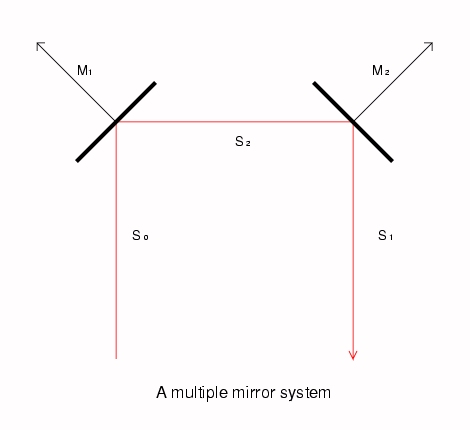`Click on any image to get the PostScript source.`
and it is also convenient to introduce a new variable ρ which will equal Γ/-2. Thus using a property of the dot product we have:
`	Γi  =  -2(Si-1∙Mi)  =  -2ρi`
In our case since we are only dealing with one mirror we can let i = 1
`	S1 = S0 + Γ1M1`
and
`	ρ1 = S0∙M1`
Since the ray is horizontal and is a unit vector we will let S0 = (1,0), and since the desired ray is at a 45º-angle we will let S1 = (√2/2, √2/2) and M1 will be the unknown. Thus we have
`	(√2/2, √2/2) = (1,0) – 2((1,0) ∙M1)M1`
or
`	(√2/2, √2/2) = (1,0) – 2(Mx)M1`
If we split the vector equation into two that involve only scalar values we will get
`	√2/2 = 1 – 2Mx2`
`	√2/2 = 0 – MxMy`
thus
`	Mx = √((√2/2-1)/2) = .382     `
and
`	My = -√2/(4Mx) = -.923`
We can also find the angle of the mirror
`	a = -tan-1(Mx/My) = -tan-1(.382/-.923) = 22.5º`
which is intuitively the right answer. //

#### Image Orientation

A useful property of the multiple mirror systems can be derived using the equation
`	Si = Si-1+2(Si-1∙Mi)Mi`
Of course 2d and 3d models are completely analogous, here the 3d one will be used. First we can see that if we split up the vectors into their individual components,
`	Six = S(i-1)x+2Mix(S(i-1)xMix+S(i-1)xMiy+S(i-1)zMiz)`
`	Siy = S(i-1)y+2Miy(S(i-1)xMix+S(i-1)xMiy+S(i-1)zMiz)`
`	Siz = S(i-1)z+2Miz(S(i-1)xMix+S(i-1)xMiy+S(i-1)zMiz)`
the equation can be written in a matrix form. Specifically:
```	| Six | 		| (1-Mix2)	-2MixMiy		-2MixMiz  || S(i-1)x|
| Siy |     = 	| -2MixMiy	(1-Miy2)		-2MiyMiz  || S(i-1)y|
| Siz | 		| -2MixMiz	-2MiyMiz		(1-Miz2)	 || S(i-1)z|```
We can try to find the determinant of the matrix, for convenience, in the following calculations Mix, Miy and Miz will be referred to as x, y, z respectively:
```	D = (1-2x2)((1-2y2)(1-2z2)-4y2z2)
+2xy(-1(1-2z2)2xy - 4yz2x)
- 2xq((4xy2z+2xz(1-2y2)```

```	D = (1-2x2)(1-2y2-2z2+4y2z2-4y2z2)
-2xy(2xy-4xyz2+4yz2x)
-2xz(4xy2z+2xz-4xzy2)```

`	D = 1 - 2y2-2z2-2x2+4x2y2+4x2z2-4x2y2-4x2z2`

`	D = 1 - 2(x2+y2+z2)`
since M is a unit vector
`	x2+y2+z2 = 1`
and
`	D = -1`
If the system involves many mirrors then the transformation has to be applied to the vector multiple times. If we let the above mentioned matrix be Ri, then:
`	(Sn) = [Rn][Rn-1]...[R1](S0)`
Thus we can see that the determinant of the matrix for the whole system is going to equal -1 if there are an odd number of matrices that are multiplied, i.e. and odd number of mirrors and it is going to equal 1 if the number of mirrors is even. The interpretation of this is that the image produced by an even number of mirrors is right handed i.e. "R" and an image produced by an even number of mirrors is left handed i.e. "Я". If the right-handed image is plain text, the left handed one is unreadable. This is a key principle in the design of prisms.

#### Some Examples of Prisms

Let's look at some examples of some simple prisms One example is Right angle prism, which produces a left-handed image and turns the angle of the rays by 90º (see picture).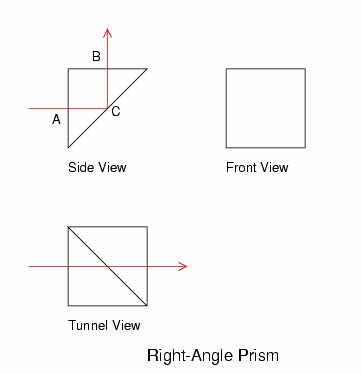If the front side is of length A then the C will be of length 1.414A. It can also be used to reverse an image i.e. turn it 180º(see picture).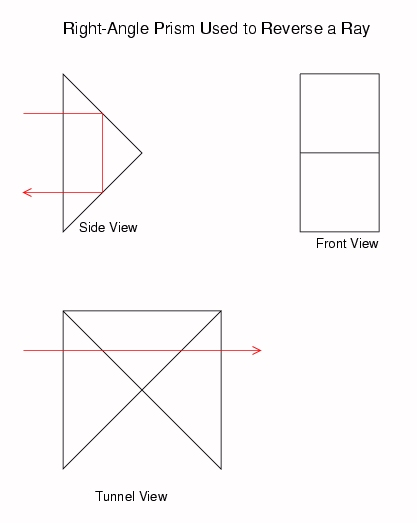In this case it will produce a left-handed image. Another example is the Dove prism. It is used to invert an image without changing its direction (see picture).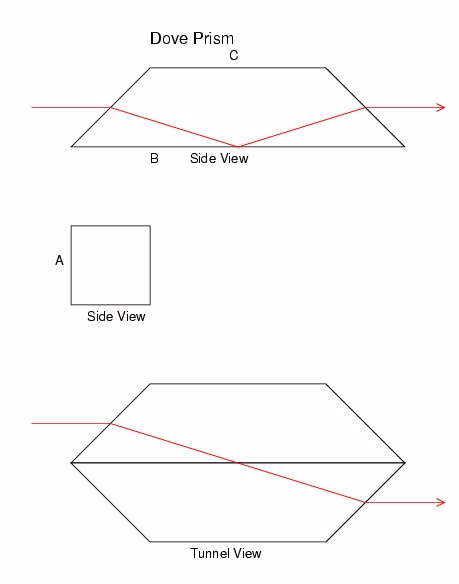##### Example 2
Find the dimensions of the Dove prism.
##### Solution:
Let the height of the front side equal A. Then the front side is at an angle of 45º. The ray entering the top the front side will have to travel to the bottom of the back side. It incident angle is 45º and the refracted angle will be
`	r = sin-1(sin(45º)/n)`
it's angle to the horizontal is
`	a = sin-1(sin(45º)/n) - 45º`
now we can find the length of side B. A is added to account for the offset of the top corner.
`	B = A tan(sin-1(sin(45º)/n) - 45º)+A`
if we let n equal 1.5170, then
`	B = 4.227A`
and
`	C = 4.227A - 2A = 2.227A`
Note that the prisms symmetry implies that the rays hitting the bottom of the front side will hit the top of the back side. //

Is it possible to make a prism with the same effect as the Dove prism but more compact? In other words how can the size to aperture ration be reduced? If the prism is rotated around the central ray by 180 degrees it will produce the same image. Thus two Dove prism with their respective side B's placed together will produce an image twice the size, but it will retain it's original length. Note that the rays that hit the bottom and the top of the prism will take opposite paths. The only remaining concern is that the rays that are refracted by the bottom side of each prism will produce a double image. This is remedied by silvering the two bottom sides, which insures that no rays pass through.
Another prism that has the same effect as the Dove prism is the Pechan Prism (see Picture). It has an advantage of that it can be placed in diverging of converging light.
As noted above the right angle prism produces a left image. If however the same angle change is required, but a right handed image is needed, a Pentaprism can be used (see picture).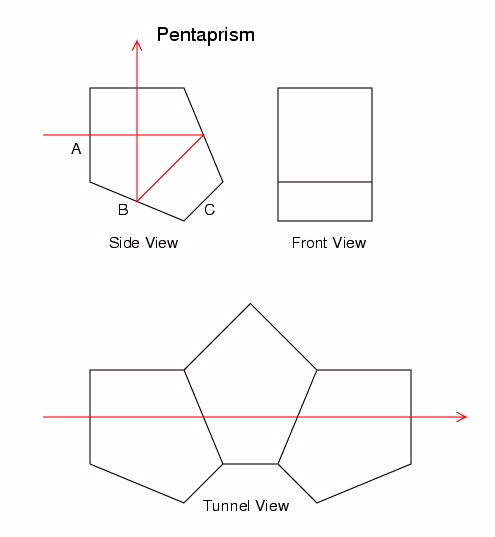##### Example 3
Find the dimensions of the Pentaprism.
##### Solution
It is obvious that side A and the top side are going to be of the same length. As we have discovered earlier the angle that a reflective surface needs to be placed at to attain a 45º angle change of ray direction is 22.5º. If we are guided by the right triangles that consist of the sides of the prism and the ray passing the front side at its bottom, we can find side B:
`	B = 1/cos(22.5º)A = 1.08239A`
we can also find side c:
`	x = tan(22.5º)A`
and
`	C = √(2(tan(22.5º)A)2) = .5857A`
Notice that unlike the Dove prism, the dimensions of the Pentaprism are independent of the value of n.//

#### Rotators

Let's go back to dove prisms. If we rotate an image along an axis of the central ray, then we know that at each point in time the top of the initial image will correspond to the bottom of the final image. I.e. a point on the initial image of height sin(Ri) will correspond to a point of height -sin(Ri) of the final image, where i is the angle of rotation. Since the two images are initially offset by 180 degrees we can also say that initial height sin(Ri) corresponds to a final height of sin(Ri+180). We can define
`	Rp = 180º + Ri`
where Rp is the rotation of the image produced by the prism and Riis the initial Rotation If instead we keep the object stationary and rotate the prism, then both the changes in Ri and Rp will be with respect to the prism. With respect to the initial image, both changes will occur. Thus the effect on the final image due to the rotation of the prism will be
`	Rf = Ri + Rp = 2Ri+180`
Where Ri is the rotation of the prism. Taking the derivative with respect to Ri we get
`	dRf = 2dRi`
This implies that the final image is rotated at twice the speed of the rotation of the prism. For this reason it is called a rotator. Let's see how this fact can be used.

### Applications

#### Periscope

A simple periscope can be constructed using just 2 mirrors, the two mirrors will have to be static relative to each other and the only way to change the target direction is to rotate the entire periscope, which means that the observer will also have to rotate. If space is limited, which is quite likely if it is used in a military setting like a trench, this might not be very practical. A better solution is achieved using the dove prism (see picture).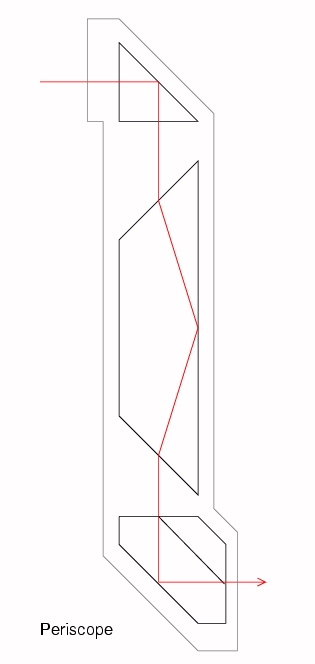A ray first passes through a right-angle prism, then a Dove prism, and then an Almici roof prism (a right-angle prism cannot be used at the bottom, because that would mean that the ray will be reflected 3 times and thus the final image will be left-handed). Now the top prism can be rotated while the bottom one remains fixed. How should the Dove prism behave? Since it rotates an image at twice the rate of the rotation of the incoming image, the Dove prism should rotate at half the angular speed of the right-angle prism. This is achieved using differential gears Keep in mind that normally a periscope would include several lenses to increase the field of view and add a magnification factor.

#### SLR Camera

An observer looking at a person taking a photo will be able to guess roughly what the image will show, simply by looking in the general direction that the camera is pointing. A photographer using a direct vision camera (one that has a viewfinder located above the main lens) has a better idea of the direction, but still cannot see exactly the image that will be produced. The most popular solution is an SLR (Single Lens Reflex) Camera (see picture),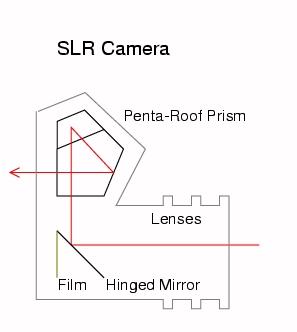which produces exactly the same image in the viewfinder and on the film. Let's look at how this is achieved. After the rays pass through the lenses, the image is both upside down and has right and left reversed. This is inconsequential with respect to the film but must be corrected by the viewfinder. Until the moment that the picture is taken, the rays are reflected at 90º by a hinged mirror. Just before the shot, the mirror swings out of the way and the rays hit the film producing the photograph. Otherwise after being reflected off the mirror the rays are traveling vertically and need to be deflected 90º towards the observer. If a right-angled prism was used for this then the resulting image would appear upside down. This can be ascertained by bouncing an imaginary pencil off the surfaces of the mirror and the prism (see picture),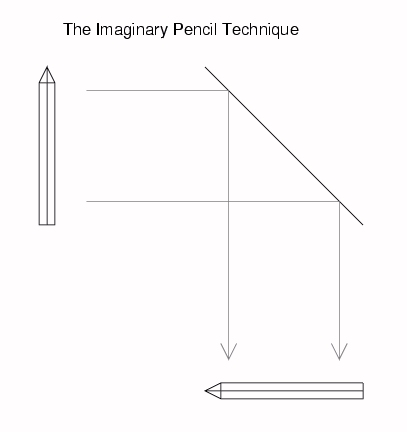keeping in mind that the image is flipped vertically when it passes through the lens. If however, a pentaprism was used the image will appear oriented the right way vertically. However there is still a problem because the image was flipped horizontally by the lens, and the lens only. To get the right horizontal orientation a penta-roof prism needs to be used. It adds one more reflection and flips the image horizontally.

### Conclusion

These are just a few samples of prisms and their applications. Many more much more complicated examples of prisms exist, and there are many interesting and useful applications of them which exploit their different properties.

### Bibliography

Dr. Robert E. Hopkins, Dr. Richard Hanau, Dr. Harold Osterberg, Dr. Oscar W. Richards, Mr. A. J. Kavanagh, Dr. Ralph Wight, Dr. Seymour Rosin, Dr. Philip Baumeister, Mr. Alva Bennet, Military Standardization Handbook, Optical Design, Defence Supply Agency, Washington, 5 October 1962.
Michael Langford, Alfred A. Knopf, Master Guide to Photography, Alfred A. Knopf Inc., London, 1982.
Walter G. Driscoll, William Vaughan, Handbook of Optics, McGraw-Hill, Inc., USA, 1978.
Rudolf Kingslake, Optical System Design, Academic Press, Inc 111 Fifth Avenue, NY, NY 10003, 1983.